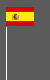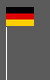# 3.1.2 mathematical modeling

We have already discussed several times the problems related to mathematical/graphical modeling. Economists in general, for the reason already mentioned, don't specify what kind of mathematics they are talking about but affirm that mathematical/graphical modeling is crucial for economics. Statement of this kind are obviously meaningless if there is no clear definition of the mathematic they talk about.

There are some sectors of economics, for instance, mathematics of finance, where mathematics is obviously crucial. Statistics, especially descriptive statistic, can be useful. It is seldom useful for proofing something because descriptive statistics doesn't explain any causal relationship, it just suggest a plausible relationship between two parameters, but can give a hint for further studies.

If we talk about mathematics in this chapter we refer to mathematical/graphical modeling.

- The use of mathematical/graphical modeling suggests that precise prognostics of the economic development is possible. If this were the case, we would not need a market economy. The strength of a market economy consists in the fact that a market economy is more efficient in dealing with insecurity. Decentral information processing through prices allows a faster adaption to changes in the economic structure than central planning. If it is impossible to predict the future, we need a system that allows to correct errors based on wrong predictions. If it were possible to predict the future with some mathematical equations, as assumed by Léon Walras, central planning would be much better. Unfortunately, this is not the world we are living in. It is not casual that in mathematical/graphical modeling we have no decision-making subjects and that capital and labour is located automatically without effort. This is the result of the methodological approach. The same is valid for the assumed transparency in this models. Transparency is not a simplification, which makes it easier to understand a crucial point of reality. The assumed transparency eliminates the fundamental problem. The underlying problem does not form part of the model. A model that doesn't contain the fundamental problem is not useful. The primary problem is a lack of transparency and incomplete information. This is the problem resolved best by a market economy. If we exclude the problem, a free market economy resolves an inexistent problem.

- Mathematical/graphical modeling is only possible if the relationship between the parameters taken into account are stable. Mathematical / graphical modeling tends, therefore, to abstract from any individual circumstances and anything unpredictable. That leads to the mathematical/graphical modeling of trivialities. A triviality of this kind is the supply curve. It is obvious that the supply increases if a higher market price allows less efficient producers to enter the market. There is no mathematical function needed to explain that. However, what we really want to know is what will happen in the long run, because, over the long term, prices decrease, see equilibrium in the short and the long run. Mathematical/graphical modeling focuses on the short term, because this is trivial and, therefore, mathematical modeling possible.

- Mathematical/graphical modeling leads to a narrow perspective. It leads to thinking that focuses more on the understanding of the model than of reality. It obliges from abstracting from relevant issues. Normal people very often have a broader view on economic problems than economists, who are trained to abstract from relevant issues.

Keynes describes it this way.

 The object of our analysis is, not to provide a machine, or methode of blind manipulation, which will furnish infallible answer, but to provide ourselves with an organised and orderly method of thinking out particular problems; and, after we have reached a provisional conclusion by isolating the complicating factors one by one, we the have to go back on ourselves an allow, as well as we can, for the probable interactions of the factors amongst themselves. This is the nature of economic thinking. Any other way of applying our formal principles of thought (without which, however, we shall be lost in de wood) will lead uns into error. It is a great fault of symbolic pseudo-mathematical methods of formalising a system of economic analysis,..., that they expressly assume strict independence between the factors involved and lose all their cogency and authority if this hypothesis is disallowed, whereas, in ordinary discourse, where we are not blindly manipulating but know all the time what we are doing and what the words mean, we can keep 'at the back of our heads' the necessary reserves an qualifications and the adjustments which we shall to make later on, in a way in which we cannot keep complicated partial differentials 'at the back' of several pages of algebra which assume that they all vanish. Too large a proposition of recent 'mathematical' economics are merely concoctions, as imprecise as the initial assumptions they rest on, which allow the author to lose sight of the complexities an interdependencies of the real world in a maze of pretensions an unhelpful symbols. John Maynard Keynes, The general Theory on Employement, Interest and money, page 148 (chapter 21, III)

We don't say that it is always a good idea to rely blindly on authorities. However, if the founder of macroeconomics, professor of economics and mathematics at the University of Cambridge questions the relevance of mathematical modeling and if there is a strong evidence for his arguments, it 's hard to ignore his statements.

There is as well strong evidence that most of the errors we find in textbooks related to the Keynesian theory are due to the mathematical and graphical presentation of his theory in the form of the IS-LM model. This model is exactly what Keynes called a "... a machine, or method of blind manipulation, which will furnish infallible answer...". While Keynes considered his theory as an instrument helping to analyse problems, the IS-LM model suggests that every problem on earth could be resolved by moving curves from the right to the left and from the left to the right. People move the IS curve to the left, increasing the aggregate demand, national income, and employment. If in reality doesn't happen what works perfectly on paper, they say that the Keynesian theory is wrong. The fact is, the Keynesian theory is right, but the IS-LM model is wrong.

The IS-LM model is as well responsible for the widespread idea that Keynesian theory is about economic management and only valid in the short run. That' completely wrong. Keynesian theory is a fundamental refutation of basic classic and neoclassic concepts about capital, interest rates, saving, and money.

The presentation of the Keynesian theory through the IS-LM model is the most illustrative example of the failure of mathematical/graphical modeling and the devastating effects of this methodological approach. Millions of people were trained on this system, and the only thing they understood is that Keynesian theory is about expansive fiscal policy. The expansive fiscal policy is one possible conclusion that can be drawn from Keynesian theory, but the crucial thing is the theoretical framework.

Due to the logic of the model, some aspects become relevant, which are entirely irrelevant. The crowding out effect, due to lack of money, doesn't exist in reality. No central bank will stop growth through a restrictive monetary policy unless they fear inflation. However, inflation has to do with the supply side, and the supply side is not part of the model.

Economists are obsessed by mathematical, graphical modeling. Although Keynes himself criticized the IS-LM model, it is presented as the authentic interpretation of the Keynesian theory. We find it in any textbook about macroeconomics.

The crucial point of Keynesian theory, the refutation of the classic concepts of capital, interest rate, saving, and money can not be modeled mathematically and are therefore excluded. That is mad.

All the mathematical and graphical models we find nowadays in textbooks about microeconomics have their origin in Principles of Economics from Alfred Marshall. That's why Alfred Marshall is considered the founder of this methodological approach. Actually, we find a mathematical description only in the annotations and Alfred Marshall was very critical concerning the use of mathematics in economics. That's the reason he ignored León Walras.

We can read everywhere that mathematics is a much more precise language than a natural language. The author would say it depends on the person who is speaking. However, apart from that mathematical modeling is blind concerning casual relationships. Casual relationships are not even needed for mathematical modeling. In any books about algebra or analysis, we find equations and functions without any context. Mathematical modeling can describe the casual relationship, but it never produce it. Mathematical modeling is as good as the theory of the mathematical modeling.

The Marxian concept surplus value = m/v * 100 (m=value added, v=variable capital) doesn't become true ore more exact because it is formulated in the form of an equation. If every relevant parameter is included in the mathematical model, a system of equations or a function, and if the relationship between these parameters is stable, we can indeed predict what will happen if one of these parameters changes. The problem is that the relationships are unstable, and we never know which parameters have to be taken into account, and we not even know if in the future won't appear new parameters to be taken into consideration. That works on a sheet of paper by using the ceteris paribus clause, but never in reality. The ceteris paribus clause excludes the fundamental problem of the economy. Insecurity and imperfect information. A model that eliminates the central problem is as useless as excluding in microbiology the existence of the virus.

We can't say why the mathematical approach becomes the methodological paradigm of economics, but it is to assume that the success of physics, where the mathematical approach is of course very useful because the relationships between the parameters are stable, plays a role in that. Using the same methods as physics economics appears to be an "exact" science. We can see or better said we could, because Marxism disappeared, this tendency in both dominant ideologies during the cold war. Ideologies never consider themselves as a mere opinion. They consider themselves as a scientifically proven truth.

This is not trivial. The methodological approach has an impact on the concepts. Many people consider neoclassic theory for instance as the contemporary practice. It is not very clear what people understand by neoclassic theory, we will discuss this topic later, see neoclassic theory, but it is to assume that they understand by neoclassic theory a free market economy, where every individual tries to get the optimal benefit of his resources and an economy which tends to general equilibrium. The neoclassic theory is considered the opponent of Marxism.

The truth it, that neoclassic theory and Marxism are very similar, because their methodological approach is very similar. Both regard the economy as the universe, with eternally valid laws, where are no subjects, no decision making, no change in fundamental structures, nothing spontaneous and nothing individual. In both systems, there are no entrepreneurs. As the water flows downwards by gravity, the capital flows to the optimal use attracted by profitability. This is a world that can be described by mathematical modeling. Unfortunately, it is not our world. In our world the use of capital, actually of money, it the result of complex, very often wrong, decision making in unclear situations with incomplete information.

notesMathematical modeling contradicts the fundamental concept of a market economy

Mathematical or graphical modeling tends to exclude everything from the model what can not be modeled with this approach

The methodological approach has an impact on the things taken into account